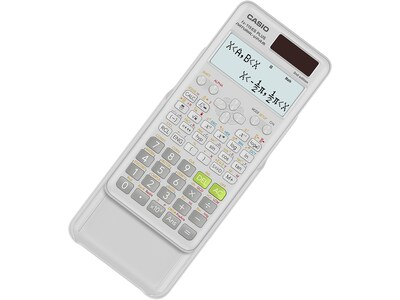# Casio 2nd Edition FX-115ESPLS2-S 16-Digit Scientific Calculator, White

Get free shipping when you order Casio 2nd Edition FX-115ESPLS2-S 16-Digit Scientific Calculator, White today at Quill.com. Stack coupons to get free gifts & extra discounts!
Item # 901-24431368
Model # FX-115ESPLS2-S\$18.99 Each

#### Qty:

This item ships FREE *
Your delivery dates will be verified at checkout.
Delivery Date:

Enter Zip Code
Product Information
Description
Casio 2nd Edition FX-115ESPLS2-S is an advanced solar-plus-battery scientific calculator that provides long life and dependability. With Natural Textbook Display, in addition to advance statistics, matrix, and conversion functionality, it's a perfect choice for high school and college students. Recommended for students taking general math, pre-algebra, algebra I and II, geometry, trigonometry, statistics, calculus, chemistry, and engineering.
• Scientific calculator with two-line Natural Textbook Display displays formulas and results simultaneously
• Perfect choice for high school and college students taking general math, pre-algebra, algebra I and II, geometry, trigonometry, statistics, calculus, chemistry, engineering
• Dimensions: 0.44"H x 3.15"W x 6.38"D
• Calculator is solar cell-powered
• 417 built-in functions
• Performs the following: addition, subtraction, multiplication, division, augmentation, absolute value, reciprocal, square, cube, and conjugate of complex number calculations
• Features linear regression, quadratic regression, logarithmic regression, e-exponential regression, ab-exponential regression, power regression, and inverse regression
• Can transform a displayed decimal calculation result to a fraction or mixed number
• Fraction/decimal conversions, simplifying fractions and roots, recurring decimal display, remainder calculation, integer division with remainder
• Prime factorization, metric conversion, hyperbolic functions, greatest common divisor and least common multiple, random integer generator, summation notation, verify computations
• Solves simultaneous linear equations with two unknowns, simultaneous linear equations with three unknowns, quadratic equations, and cubic equations
• Matrix mode allows you to perform matrix operations on matrices with up to three rows and three columns, also allowing you to enter vectors and perform vector calculations
• Features a new sleek design and hard slide-on case
• 1-year manufacturer limited warranty
Happy to help1-800-982-3400